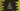# C# program to print the Floyd's triangle## C# program to print the Floyd’s triangle:

Floyd’s triangle is named after Robert Floyd. This is a triangle that is created by filled all rows in the triangle with consecutive numbers. For example, below is a Floyd’s triangle of 5 rows:

``````1
2  3
4  5  6
7  8  9  10
11 12 13 14 15``````

In this post, we will write one C# program that will print the Floyd’s triangle. This program will print the triangle for any number or rows.

### Algorithm to print the Floyd’s triangle:

We need to use two loops to print this triangle. The outer loop will run for row number of times and the inner loop will run to print the values.

• Run one loop from 1 to number of rows.
• Inside each iteration of the loop, run another loop. That will run for current iteration value number of times. For the first row, it will run for one time, for the second row, it will run for two times etc.
• This inner loop will print the values of the triangle. For that, we will create one variable initialized with 1. After each print statement, we will increment the value by 1.

### Floyd’s triangle C# program:

Below is the complete C# program that prints a Floyd’s triangle:

``````using System;

namespace c_sharp
{
public class Program
{
public static void Main(string[] args)
{
int height, i, j;
int value = 1;
String triangleValue;

Console.WriteLine("Enter the height of the triangle :");
height = Convert.ToInt32(Console.ReadLine());
Console.WriteLine();

for (i = 1; i<= height; i++){
for(j = 1; j< i+1; j++){
triangleValue = value > 9 ? (value + "") : (value + " ");

Console.Write(triangleValue +" ");
value++;
}
Console.WriteLine();
}

}
}
}``````

Here,

• height is to store the height of the triangle. i and j are to use in the for loops.
• value is the value to print in the triangle.
• This program first takes the height of the triangle as an input from the user. It reads the input, converts it to integer and stores it in height.
• The outer for loop runs from i = 1 to i = height. This loop is for each rows of the triangle. The inner for loop runs from j = 1 to j = i. This loop is to print each value in the triangle.
• triangleValue is a variable to hold the value to print in the triangle. If the value is smaller than 9, then it adds one blank space to end of this value to match the spacings.
• It prints the triangleValue and adds one space to its end to separate each values in the triangle. Then, it increments the value by 1.
• Once the iteration of the inner loop ends, it prints one new-line. This will move the control to the next line.

If you run this program, it will print the below output:

``````Enter the height of the triangle :
5

1
2  3
4  5  6
7  8  9  10
11 12 13 14 15

Enter the height of the triangle :
10

1
2  3
4  5  6
7  8  9  10
11 12 13 14 15
16 17 18 19 20 21
22 23 24 25 26 27 28
29 30 31 32 33 34 35 36
37 38 39 40 41 42 43 44 45
46 47 48 49 50 51 52 53 54 55``````

As you can see here, it takes the height of the triangle and prints it.